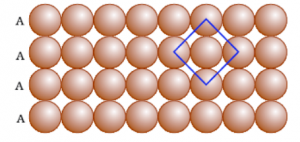# Packing In Solids - One And Two Dimensions

Crystalline solids exhibit a regular and repeating pattern of constituent particles. The diagrammatic representation of three-dimensional arrangements of constituent particles in a crystal, in which each particle is depicted as a point in space is known as crystal lattice. In a crystal lattice, the atoms are very closely packed leaving very little space between them. Packing in solids in different dimensions is discussed below:

### Close packing in crystalline solids in one dimension:

In one dimension close packing, spheres are arranged in a row such that adjacent atoms are in contact with each other. Coordination number is defined as the no. of the nearest neighbour particles. In case of one dimension close packing, coordination number is equal to two.### Close packing in crystalline solids in two dimension:

In two-dimensional close packing, row of closed packed spheres are stacked to obtain a two-dimensional pattern. This stacking is done in two ways:

• Square close packing: The second row can be placed exactly below the first row in a close packing. Thus, if we call the first row as “A” type row, the second row being arranged exactly the same as the first one, is also of “A” type. In such an arrangement each sphere is in contact with four other spheres. Hence, it has a coordination number equal to four. We observe that if the centres of the four immediate neighbouring spheres are joined, a square is formed. This type of packing in crystalline solids is known as square close packing in two dimensions.Packing in Solids: One and Two Dimensions

• Hexagonal close packing: The second row can be placed below the first row in a staggered manner such that its spheres fit in the depressions of first row. Thus, if we call the first row as “A” type row, the second row being arranged differently can be named as “B” type. Again the third row appears as “A” type. This type of packing is referred as “ABAB” type. In such an arrangement each sphere is in contact with six other spheres. Hence, it has a coordination number equal to six. We observe that if the centres of the six immediate neighbouring spheres are joined, a hexagon is formed. This type of packing in solids is known as hexagonal close packing in two dimensions. It has lesser free space and hence higher packing efficiency in comparison to square close packing.For a detailed discussion on packing in crystalline solids in one and two dimensions, download BYJU’S – the learning app.